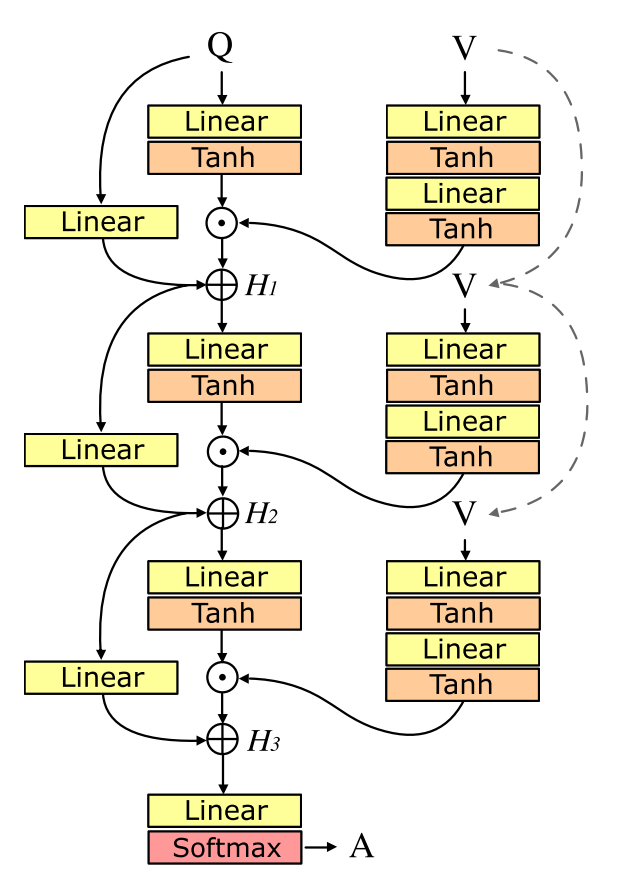# Multimodal Residual Learning for Visual QA

## WHY?

Visual question answering task is to answer to natural language question based on images requiring extraction of information from both images and texts. Stacked Attention Networks(SAN) stacked several layers of attention to answer to complicated questions that requires reasoning. Multimodal Residual Network (MRN) points out weighted averaging of attention layers in SAN works as a bottleneck restricting the information of interaction between questions and images.

## WHAT?

To address the bottleneck issue, MRN increased expressiveness of interaction between question and image by performing elementwise product instead of attention mechanism.H_0 = \mathbf{q}\\
H_1(\mathbf{q}, \mathbf{v}) = W_{\mathbf{q}'}^{(1)}\mathbf{q} + \mathcal{F}^{(1)}(\mathbf{q}, \mathbf{v})\\
\mathcal{F}^{(k)}(\mathbf{q}, \mathbf{v}) = \sigma(W^{(k)}_{\mathbf{q}}\mathbf{q})\odot\sigma(W_2^{(k)}\sigma(W_1^{(k)}\mathbf{v}))\\
H_L(\mathbf{q}, \mathbf{v}) =  W_{\mathbf{q}'}\mathbf{q} + \sum^L_{l=1}W_{\mathcal{F}^{(l)}}\mathcal{F}^{(l)}(H_{l-1}, \mathbf{v})\\
W_{\mathbf{q}'} = \prod^L_{l=1}W^{(l)}_{\mathbf{q}'}\\
W_{\mathcal{F}^{(l)}} = \prod^L_{m=l+1} W^{(m)}_{\mathbf{q}'}

## So?

NRM achieved state-of-the-art result in open-ended and multiple-choice of VQA dataset.

## Critic

There may be more sophisticated way to decide the level of logic instead of just stacking numerous layers.

Powered by aiden